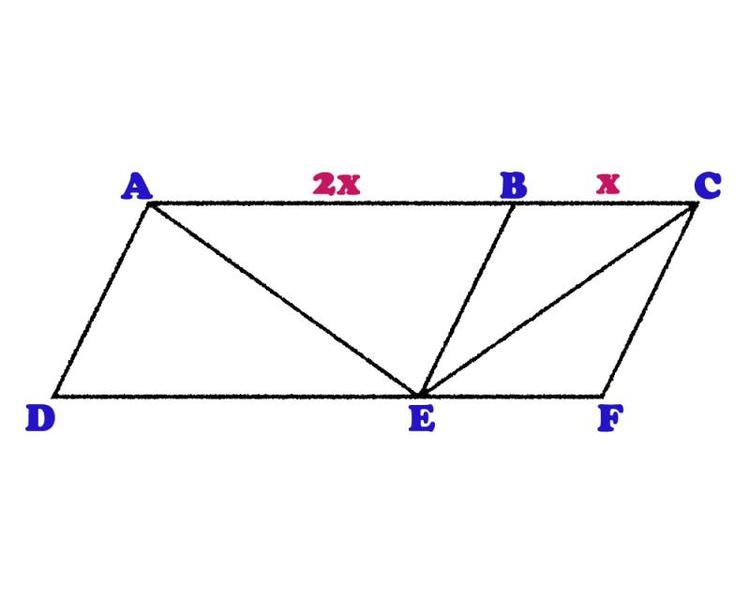# Starting the Sander's Parallelogram Proof

Can anyone help me set this problem up?

I am trying to figure out how to Prove the Sander's Parallelogram.

See it here:
http://www.tigel.nl/fun/files/opticals/Ill...ith_line-25.htm [Broken]

basically it is proving that the bisectors are of equal length

The question is: what would be needed to be established first in order to properly put together a proof? Are they a specific size? is there a relationship between the length of the two top sides? Any help would be great.

I don't need the proof - I am certain I can figure it out, but I would like a leg up on figuring out what would need to be established before starting to work it out.

many thanks!

Last edited by a moderator:
•JamesMaths

I think I should clarify what I am trying to do. I do not wish to construct the parallelogram. I want to be able to set up the initial conditions by which I could then ask someone to prove that the two bisectors are equal. By this I mean a standard two column mathematical proof.

I do know, after playing around that, as you can see from the image below, that AB is twice as big as BC. but beyond that, what else would one need to know?

many thanks with the help so far.pbuk
Gold Member
I do not wish to construct the parallelogram. I want to be able to set up the initial conditions by which I could then ask someone to prove that the two bisectors are equal.

But these two problems are equivalent - if you know how to construct the parallelogram you know what needs to be specified that means that the two diagonals are equal.

I do know, after playing around that, as you can see from the image below, that AB is twice as big as BC.

That may be true for the example you have chosen, but it is not a necessary condition for the two diagonals to be equal - do an image search for Sander's Parallelogram and you will see examples of all sorts of shapes.

So what rules do all such parallelograms follow? The best way to find out is to try constructing one yourself, either starting with any equilateral triangle and constructing a parallelogram around it, or starting with any parallelogram and constrcting an equilateral triangle within it. Because you can do this whatever shape you start off with, the only "initial conditions" that are sufficient to specify a Sander Parallelogram are that the two diagonals are equal! I'm afraid your quest for something to "prove" is futile.